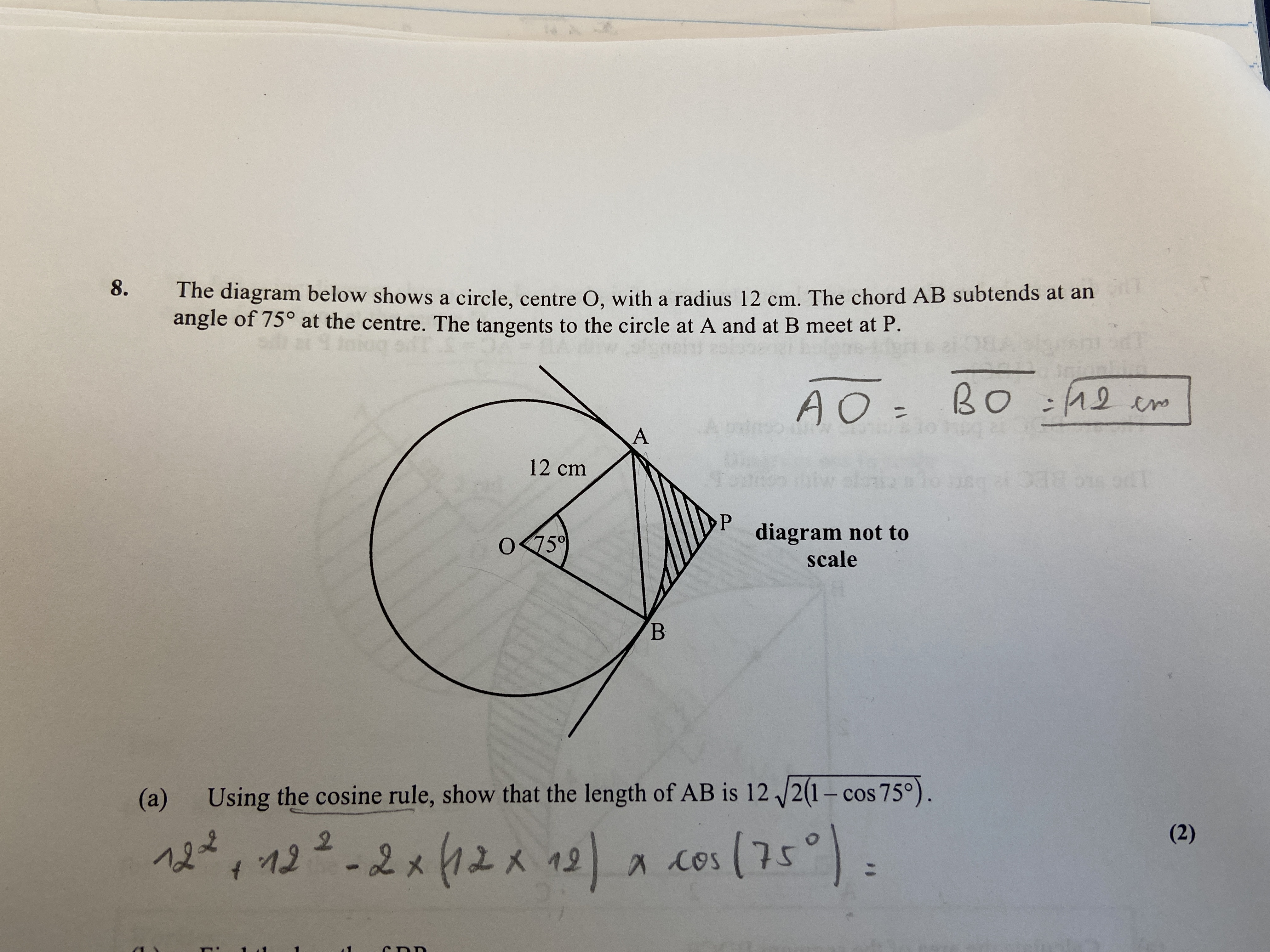# Help Zone

### Student Question

Bonsoir, j'ai du mal à faire cet exercice aussi.

Merci.Mathematics## Explanations (1)

•Explanation from AlloprofExplanation from Alloprof

This Explanation was submitted by a member of the Alloprof team.

Hello,

You were on the right track!

Indeed, the cosine formula you used is the right one and the numbers you put are correct as well. All you had to do was continue to calculate and reduce the expression, for example by factoring out.

Don't forget the other side of the equality. 😉

\begin{align} c^2 &= 12^2 + 12^2 - 2(12)(12)cos75^{o}\\ c^2 &= 2(12)^2 - 2(12)^2 cos75^{o} \\ c^2 &= 2(12)^2 ( 1 - cos75^{o} )\\ c &= \sqrt{ 2(12)^2 ( 1 - cos75^{o} )} \\ c &= \sqrt{ (12)^2)} \sqrt{2 ( 1 - cos75^{o} )} \\ c &= 12 \sqrt{2 ( 1 - cos75^{o} )} \\ \end{align}

Here is more details about the cosine rule in case you need it!

Here are more examples on how to factor out.

Don't hesitate to ask other questions!